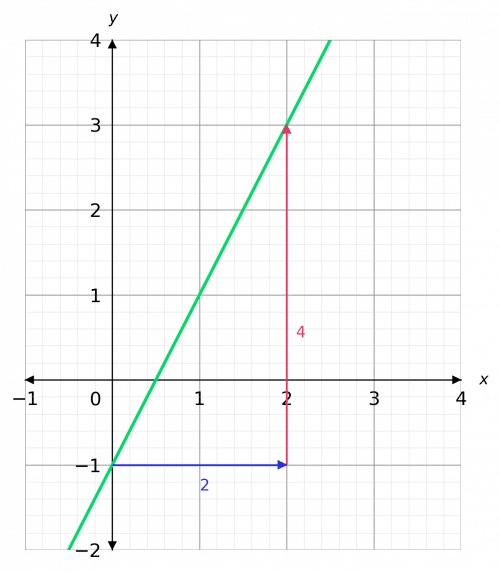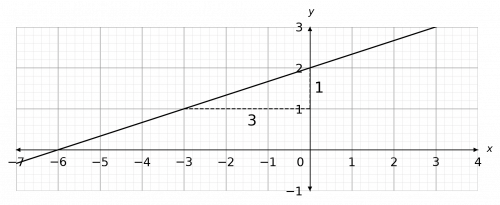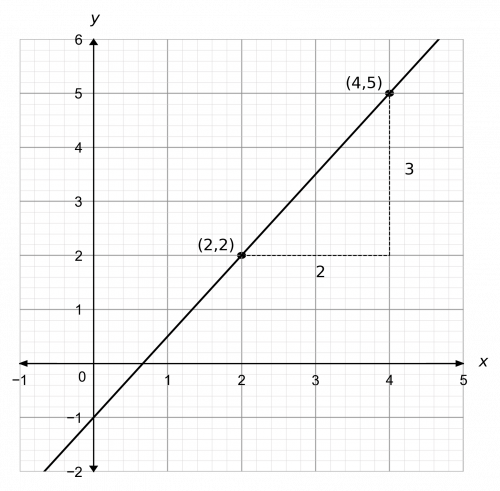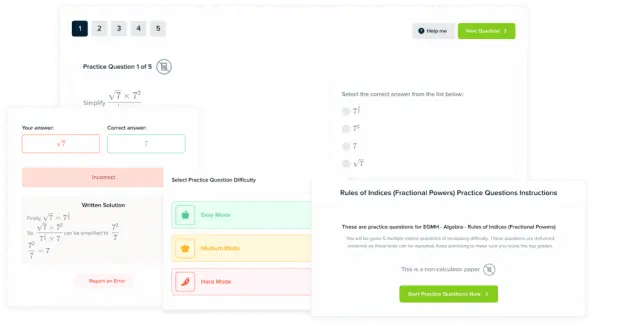# y=mx+c

GCSEKS3Level 4-5AQAEdexcelEdexcel iGCSEOCRWJEC

## The Equation of a Straight Line ($y=mx+c$)

A straight line graph will always have an equation in the form $y=mx+c$. You need to be able to work out the equation of a straight line from a graph, as well as manipulate the equation itself.

There are 3 key skills you need to learn involving the equation of a straight line.

Make sure you are familiar with the following topics before continuing.

## The Straight Line Equation

Any straight line graph can be described  by the following equation:

$\textcolor{red}{y}=\textcolor{limegreen}{m}\textcolor{red}{x}+\textcolor{blue}{c}$

where $\textcolor{red}x$ and $\textcolor{red}y$ are the coordinates the line passes through, $\textcolor{limegreen}m$ is the gradient and $\textcolor{blue}c$ is the $y$-intercept (the $y$-coordinate where the line crosses the $y$ axis).

Level 4-5GCSEKS3AQAEdexcelOCRWJECEdexcel iGCSE
Level 4-5GCSEKS3AQAEdexcelOCRWJECEdexcel iGCSE

## Skill 1: Finding the Equation of a Straight Line

We need to be able to find the equation of a straight line from the graph.

Example: Find the equation of the straight line graph belowStep 1: Find $\textcolor{blue}{c}$

We are looking for an equation of the form,

$y=\textcolor{limegreen}{m}x+\textcolor{blue}{c}$

We know $\textcolor{blue}{c} = y$-intercept. Looking at the graph, we can see it crosses the axis at $- 1$, therefore we have $\textcolor{blue}{c=-1}$.Step 2: Find the gradient ($\textcolor{limegreen}{m}$)

Then, to work out the gradient,

$\text{Gradient } = \dfrac{\textcolor{red}{\text{change in }y}}{\textcolor{blue}{\text{change in }x}}$

The triangle we have drawn has height $4$ and width $2$, so we get,

$m=\text{gradient}=\dfrac{\textcolor{red}{4}}{\textcolor{blue}{2}}=\textcolor{limegreen}{2}$

Therefore, the equation of the straight line is,

$y=\textcolor{limegreen}{2}x\textcolor{blue}{-1}$

Level 4-5GCSEKS3AQAEdexcelOCRWJECEdexcel iGCSE
Level 4-5GCSEKS3AQAEdexcelOCRWJECEdexcel iGCSE

## Skill 2: Finding the Equation of a Line Through Two Points

Finding the equation of a straight line between two points is an important skill.

Example: Find the equation of the line that passes through $(-3, 1)$ and $(2, -14)$.

$m=\text{gradient}=\dfrac{(-14)-1}{2-(-3)}=\dfrac{-15}{5}=-3$

Now we know that $m=-3$, we know that our equation must take the form,

$y=-3x+c$

Step 2: Substitute the $x$ and $y$ values of one co-ordinate, say $x = -3, y=1$, into the equation,

$1=(-3)\times(-3)+c=9+c$

Step 3: Rearrange to solve for $c$,

$c=1-9=-8$

Step 4: Now we have all the components of the equation of a line, we can write the resulting equation as,

$y=-3x-8$

Level 4-5GCSEKS3AQAEdexcelOCRWJECEdexcel iGCSE
Level 4-5GCSEKS3AQAEdexcelOCRWJECEdexcel iGCSE

## Skill 3: Rearranging Equations into the form $y=mx+c$

It is often necessary to rearrange the equation of a line to get it in the form $y=mx+c$. This is essential for finding the gradient and $y$-intercept.

Example: Find the gradient and $y$-intercept of the line $x+2y=14$.

We want to rearrange this equation to make $y$ the subject. So, subtracting $x$ from both sides, we get

$2y=-x+14$

Then, dividing both sides by $2$, we get

$y=-\dfrac{1}{2}x+7$

Therefore, the gradient is $-\dfrac{1}{2}$ and the $y$-intercept is $7$.

Level 4-5GCSEKS3AQAEdexcelOCRWJECEdexcel iGCSE

## y=mx+c Example Questions

We want an equation of the form

$y=mx+c$

So, we need to find the gradient, $m$, and $y$-intercept, $c$.

Firstly, looking at the graph we can see that the $y$-intercept is $2$, so $c=2$.

Now, we will find the gradient by drawing a triangle underneath the line in question.The triangle we have drawn has height $1$ and width $3$, so we get

$m=\text{gradient}=\dfrac{1}{3}$

Therefore, the equation of the line is

$y=\dfrac{1}{3}x+2$Gold Standard Education

We want an equation of the form

$y=mx+c$

So, we need to find the gradient, $m$, and $y$-intercept, $c$.

Firstly, we will find the gradient by dividing the difference in the $y$ coordinates by the difference in the $x$ coordinates:

$m=\text{gradient}=\dfrac{-6-34}{-3-2}=\dfrac{-40}{-5}=8$

Therefore, the equation of the line is

$y=8x+c$

Then, to find $c$ we will substitute one pair of coordinates that the line passes through into the equation and rearrange. Here, we’ll pick $(2, 34)$. Subbing this in, we get

$34=8\times2+c=16+c$

Subtracting $16$ from both sides, we get

$c=34-16=18$

Therefore, the equation of the line is

$y=8x+18$.Gold Standard Education

We want an equation of the form

$y=mx+c$

So, we need to find the gradient, $m$, and $y$-intercept, $c$.

Firstly, looking at the graph we can see that the $y$-intercept is $- 1$, so $c=-1$.

Now, we will find the gradient by drawing a triangle underneath the line in question. Hence

$m=\text{gradient}=\dfrac{3}{2}$Therefore, the equation of the line is

$y=\dfrac{3}{2}x-1$Gold Standard Education

## y=mx+c Worksheet and Example Questions

### (NEW) y=mx+c Exam Style Questions - MME

Level 4-5GCSEKS3NewOfficial MMEProduct

### £19.99

/month

Learn an entire GCSE course for maths, English and science on the most comprehensive online learning platform. With revision explainer videos & notes, practice questions, topic tests and full mock exams for each topic on every course, it’s easy to Learn and Revise with the MME Learning Portal.

## Related Topics

Level 4-5GCSEKS3

#### Rearranging Formulae

Level 4-5Level 6-7GCSEKS3